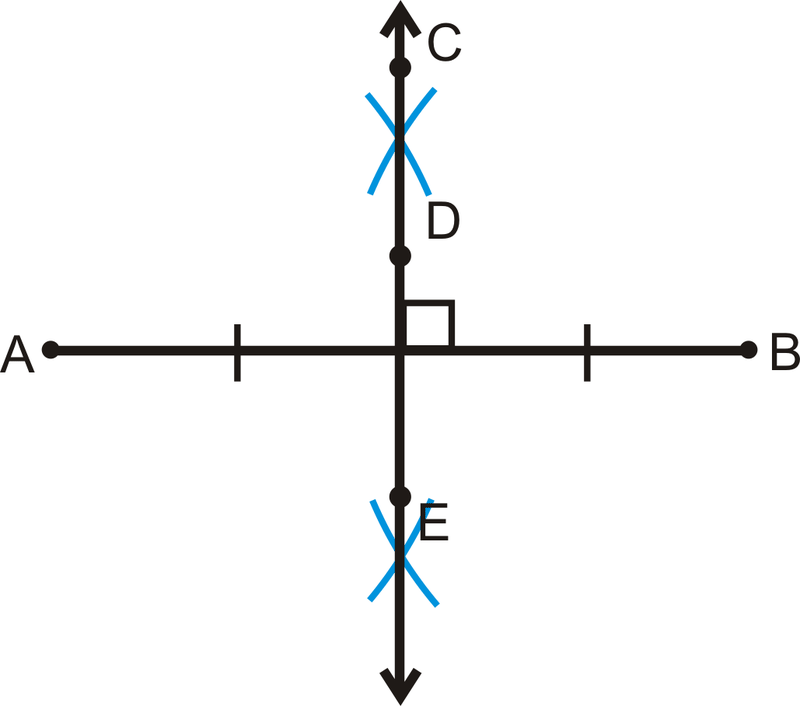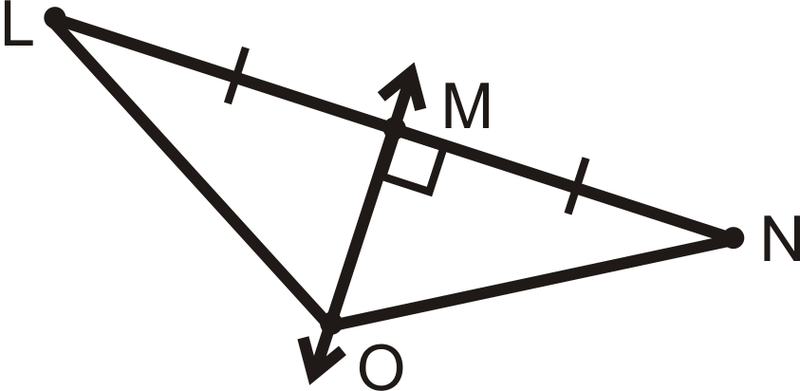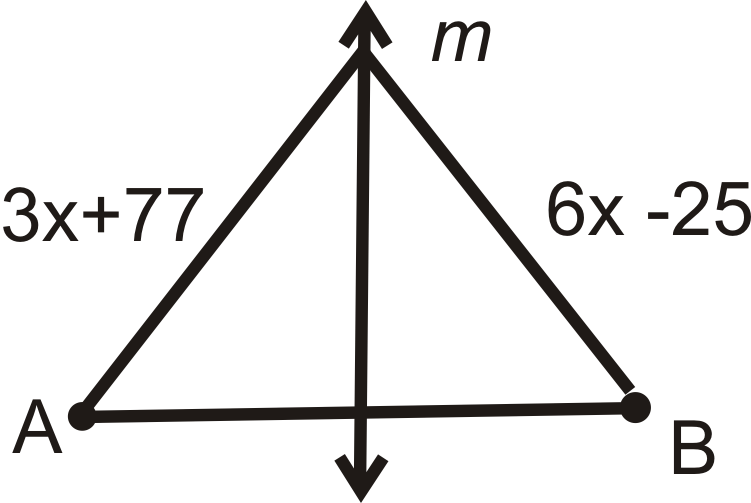# 4.20: Perpendicular Bisectors

•• Contributed by CK12
• CK12

Intersect line segments at their midpoints and form 90 degree angles with them.

## Perpendicular Bisector Theorem

A perpendicular bisector is a line that intersects a line segment at its midpoint and is perpendicular to that line segment, as shown in the construction below.Figure $$\PageIndex{1}$$

One important property related to perpendicular bisectors is that if a point is on the perpendicular bisector of a segment, then it is equidistant from the endpoints of the segment. This is called the Perpendicular Bisector Theorem.

If $$\overleftrightarrow{CD}\perp \overline{AB}$$ and $$AD=DB$$, then $$AC=CB$$.Figure $$\PageIndex{2}$$

In addition to the Perpendicular Bisector Theorem, the converse is also true.

Perpendicular Bisector Theorem Converse: If a point is equidistant from the endpoints of a segment, then the point is on the perpendicular bisector of the segment.

Using the picture above: If $$AC=CB$$, then $$\overleftrightarrow{CD}\perp \overline{AB}$$ and $$AD=DB$$.

When we construct perpendicular bisectors for the sides of a triangle, they meet in one point. This point is called the circumcenter of the triangle.Figure $$\PageIndex{3}$$

What if you were given $$\Delta FGH$$ and told that $$\overleftrightarrow{GJ}$$ was the perpendicular bisector of $$\overline{FH}$$? How could you find the length of FG given the length of GH\)?

Example $$\PageIndex{1}$$

$$\overleftrightarrow{OQ}$$ is the perpendicular bisector of $$\overline{MP}$$.Figure $$\PageIndex{4}$$

Which line segments are equal? Find $$x$$. Is $$L$$ on $$\overleftrightarrow{OQ}$$? How do you know?

Solution

$$ML=LP$$, $$MO=OP$$, and $$MQ=QP$$.

\begin{align*} 4x+3&=11 \\ 4x&=8 \\ x&=2\end{align*}

Yes, $$L$$ is on $$\overleftrightarrow{OQ}$$ because $$ML=LP$$ (the Perpendicular Bisector Theorem Converse).

Example $$\PageIndex{2}$$

Determine if $$\overleftrightarrow{ST}$$ is the perpendicular bisector of $$\overline{XY}$$. Explain why or why not.Figure $$\PageIndex{5}$$

Solution

$$\overleftrightarrow{ST}$$ is not necessarily the perpendicular bisector of $$\overline{XY}$$ because not enough information is given in the diagram. There is no way to know from the diagram if $$\overleftrightarrow{ST}$$ will extend to make a right angle with $$\overline{XY}$$.

Example $$\PageIndex{3}$$

If $$\overleftrightarrow{MO}$$− is the perpendicular bisector of $$\overline{LN}$$ and $$LO=8$$, what is $$ON$$?Figure $$\PageIndex{6}$$

Solution

By the Perpendicular Bisector Theorem, $$LO=ON$$. So, $$ON=8$$.

Example $$\PageIndex{4}$$

Find $$x$$ and the length of each segment.Figure $$\PageIndex{7}$$

Solution

$$\overleftrightarrow{WX}$$− is the perpendicular bisector of $$\overline{XZ}$$ and from the Perpendicular Bisector Theorem $$WZ=WY$$.

\begin{align*} 2x+11&=4x−5 \\ 16&=2x \\ 8&=x \end{align*}

$$WZ=WY=2(8)+11=16+11=27$$.

Example $$\PageIndex{5}$$

Find the value of $$x$$. $$m$$ is the perpendicular bisector of $$AB$$.Figure $$\PageIndex{8}$$

Solution

By the Perpendicular Bisector Theorem, both segments are equal. Set up and solve an equation.

\begin{align*}3x−8&=2x \\ x&=8 \end{align*}

## Review

For questions 1-4, find the value of $$x$$. m\) is the perpendicular bisector of$$AB$$.

1.Figure $$\PageIndex{9}$$
2.Figure $$\PageIndex{10}$$
3.Figure $$\PageIndex{11}$$
4.Figure $$\PageIndex{12}$$

m is the perpendicular bisector of $$\overline{AB}$$.Figure $$\PageIndex{13}$$
1. List all the congruent segments.
2. Is $$C$$ on $$m$$? Why or why not?
3. Is $$D$$ on $$m$$? Why or why not?

For Question 8, determine if $$\overleftrightarrow{ST}$$ is the perpendicular bisector of \overline{XY}\). Explain why or why not.

1. In what type of triangle will all perpendicular bisectors pass through vertices of the triangle?
2. Fill in the blanks of the proof of the Perpendicular Bisector Theorem.Figure $$\PageIndex{15}$$

Given: $$\overleftrightarrow{CD}$$ is the perpendicular bisector of $$\overline{AB}$$

Prove: $$\overline{AC}\cong \overline{CB}$$

Statement Reason
1. 1.
2. $$D$$ is the midpoint of $$\overline{AB}$$ 2.
3. 3. Definition of a midpoint
4. $$\angle CDA$$ and $$\angle CDB$$ are right angles 4.
5. $$\angle CDA\cong \angle CDB$$ 5.
6. 6. Reflexive PoC
7. $$\Delta CDA\cong \Delta CDB$$ 7.
8. $$\overline{AC}\cong \overline{CB}$$ 8.

### Vocabulary

Term Definition
circumcenter The circumcenter is the point of intersection of the perpendicular bisectors of the sides in a triangle.
perpendicular bisector A perpendicular bisector of a line segment passes through the midpoint of the line segment and intersects the line segment at $$90^{\circ}$$.
Perpendicular Bisector Theorem Converse If a point is equidistant from the endpoints of a segment, then the point is on the perpendicular bisector of the segment.

Interactive Element

Video: Perpendicular Bisectors Principles - Basic

Activites: Perpendicular Bisectors Discussion Questions

Study Aid: Bisectors, Medians, Altitudes Study Guide

Practice: Perpendicular Bisectors

Real World: Perpendicular Bisectors